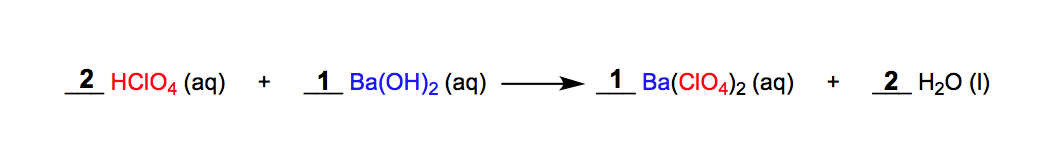Clutch Prep is now a part of Pearson
Ch.4 - Chemical Quantities & Aqueous ReactionsWorksheetSee all chapters

# Molecular Equations

See all sections
Sections
Solutions
Molarity
Osmolarity
Dilutions
Solubility Rules
Electrolytes
Molecular Equations
Gas Evolution Equations
Solution Stoichiometry
Complete Ionic Equations
Calculate Oxidation Numbers
Redox Reactions
Balancing Redox Reactions: Acidic Solutions
Balancing Redox Reactions: Basic Solutions
Activity Series

Molecular Equations show reacting molecules involved in a chemical reaction.

###### Examining Molecular Equations

Concept #1: Introduction to Molecular Equations

In a typical molecular equation, two reactants dissociate and reorganize to form new productsExample #1: Which of the following is a precipitation reaction?

Example #2: Predict whether a chemical reaction occurs and write the balanced molecular equation.

A reaction occurs only if solid, gas or liquid water is formed. If both products are aqueous, NO REACTION has occurred.

Practice: Predict whether a chemical reaction occurs and write the balanced molecular equation.

Ag2SO4 (aq) + KCl (aq) →

Practice: Predict whether a chemical reaction occurs and write the balanced molecular equation.

MgBr2 (aq) +  NaC2H3O2 (aq) →

Practice: Determine the balanced equation for the neutralization equation

Ca(OH)2 (aq) + HCN (aq) →